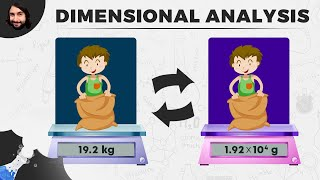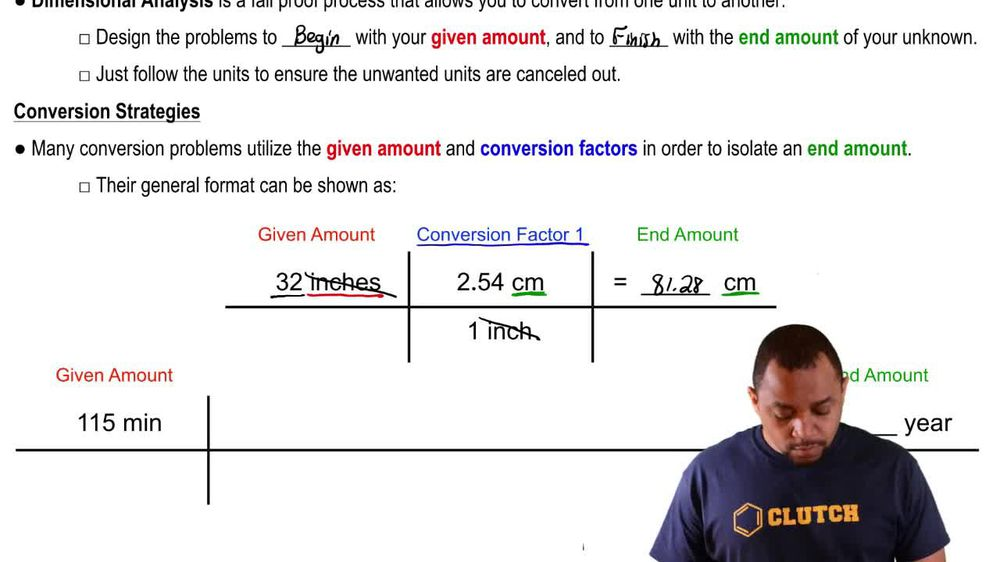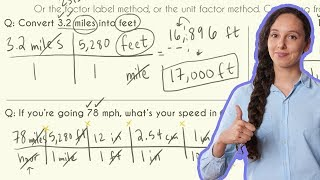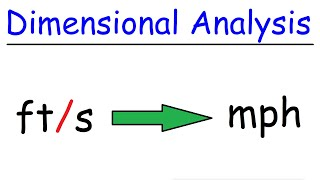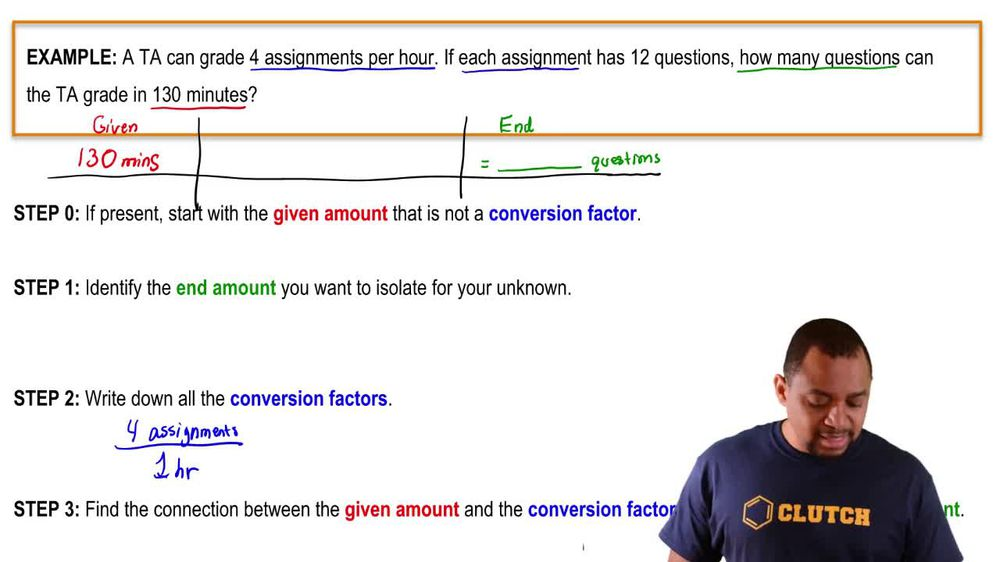Start typing, then use the up and down arrows to select an option from the list.
1. 1. Intro to General Chemistry2. Dimensional Analysis
Problem

# Convert the following quantities into SI units with the correct number of significant figures. (e) 978.3 yd3

Relevant Solution59s
Play a video:
Hi everyone today, we have a question telling us that 7400 ounces of coke fits in a soda can and our goal is to convert the units to meters cubed first. We have to remember our conversion factor. So 33, 814oz equals one m cubed. And now we need to put this in an equation. So we're going to start out with our 7400 oz of coke And we are going to multiply that by one m Cube because that is our goal unit And that is going to be over our conversion factor, which is 33,814 oz. So our answers here are going to cancel out and that is going to equal 0.22 m cubed. And that is our final answer. Thank you for watching. Bye.